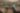# An Exploration Strategy Facing Non-Stationary Agents (JAAMAS Extended Abstract)

Date May 8, 2017
Authors P. Hernandez-Leal, Y. Zhan, M. E. Taylor, L. E. Sucar, E. Munoz de Cote

Multi-agent systems where the agents interact among themselves and with a stochastic environment can be formalized as stochastic games. We study a subclass of these games, named Markov potential games (MPGs), that appear often in economic and engineering applications when the agents share some common resource. We consider MPGs with continuous state-action variables, coupled constraints and nonconvex rewards. Previous analysis followed a variational approach that is only valid for very simple cases (convex rewards, invertible dynamics, and no coupled constraints); or considered deterministic dynamics and provided open-loop (OL) analysis, studying strategies that consist in predefined action sequences, which are not optimal for stochastic environments. We present a closed-loop (CL) analysis for MPGs and consider parametric policies that depend on the current state and where agents adapt to stochastic transitions. We provide easily verifiable, sufficient and necessary conditions for a stochastic game to be an MPG, even for complex parametric functions (e.g., deep neural networks); and show that a closed-loop Nash equilibrium (NE) can be found (or at least approximated) by solving a related optimal control problem (OCP). This is useful since solving an OCP---which is a single-objective problem---is usually much simpler than solving the original set of coupled OCPs that form the game---which is a multiobjective control problem. This is a considerable improvement over the previously standard approach for the CL analysis of MPGs, which gives no approximate solution if no NE belongs to the chosen parametric family, and which is practical only for simple parametric forms. We illustrate the theoretical contributions with an example by applying our approach to a noncooperative communications engineering game. We then solve the game with a deep reinforcement learning algorithm that learns policies that closely approximates an exact variational NE of the game.

View paper

Share
,,